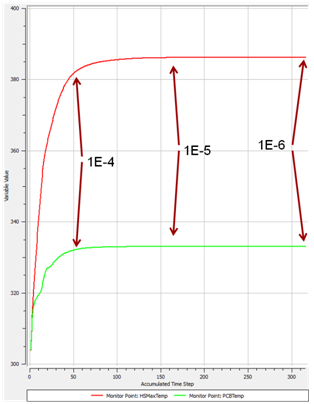# Has My CFD Analysis Converged? - Part IISeptember 12, 2014 By: Michael Kuron

In Part I of this post, I discussed the use of residual values as a measure of convergence in a steady state CFD analysis. In this post, I'll expand my discussion on convergence to the utility of solution imbalances and monitor points as a means of judging convergence.

Solution Imbalances:
Since the CFD code is solving conservation equations (conservation of mass, momentum, energy, etc.), we want to ensure that the final, converged solution does indeed conserve these quantities. As a numerical representation of a physical system, the CFD solution imbalances will never be exactly zero, however, they should be sufficiently small before considering the solution converged. A good rule of thumb is to aim for solution imbalances of less than 1% as a starting point. For the most part, having sufficiently small values of the solution residuals will lead to small solution imbalances. However, for cases that have processes with widely different timescales, it is possible to have large imbalances even when the residuals are small. A common example of this occurs in Conjugate Heat Transfer (CHT) analyses where the conduction timescales can be much longer than the flow timescales.

Monitoring of Quantities of Interest:
In a steady state CFD analysis, the solution field should no longer change from iteration to iteration in order for an analysis to be deemed converged. Monitoring integrated quantities such as force, drag, or average temperature can help the user judge when his or her analysis has reached this point. Using the heat sink example from above, two useful monitor points might be the maximum temperature of the heat sink and the volumetric average temperature in the heat generating component.In the figure above, we can see the change in the monitor point values vs. iteration number and RMS residual value. After approximately 50 iterations, the RMS residuals are reduced to 1E-4 and the average temperature monitor point is within just a few percent of its final value. As the residuals decrease further, the monitor values change less and less between iterations. Once the monitor point values have "flattened out", we can safely assume the solution is converged.

There are, of course, many other things to consider when judging the value of CFD results. Just because the solution is numerically accurate does not mean that it is a good representation of the true physical behavior. A converged solution is not very useful if it's a byproduct of incorrect boundary conditions! Any model should be thoroughly checked, from geometry and mesh to boundary conditions and solver settings to ensure its suitability for the problem at hand.